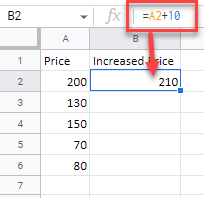# Add Values to Cells / Columns in Excel & Google Sheets

This tutorial demonstrates how to add values to cells and columns in Excel and Google Sheets.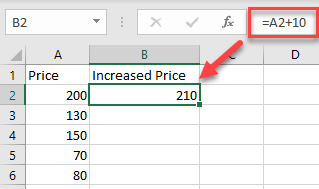## Add Values to Multiple Cells

1. To add a value to a range of cells, select the cell where you want to display the result, and enter = (equal) and the cell reference of the first number then + (plus) and the number you want to add.

For this example, start with cell A2 (200). Cell B2 shows the Price in A2 increased by 10. So, in cell B2, enter:

``=A2+10``As a result, the value in cell B2 is now 210 (value from A2 plus 10).

1. Now copy this formula down the column to the rows below. Select the cell where you entered the formula (F2) and place your cursor on the bottom right corner of the cell.
2. When it changes to a plus sign, drag it down to the rest of the rows where you want to apply the formula (here, B2:B6).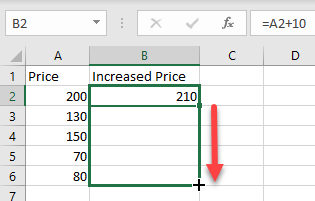As a result of relative cell referencing, the constant (here, 10) remains the same, but the cell address changes according to the row you are in (so B3=A3+10, and so on).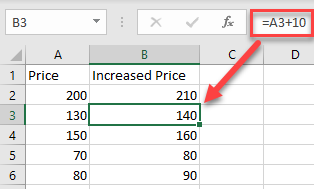## Add Multiple Cells With Paste Special

You can also add a number to multiple cells and return the result as a number in the same cell.

1. First, select the cell with the value you want to add (here, cell C2), right-click, and from the drop-down menu, choose Copy (or use the shortcut CTRL + C).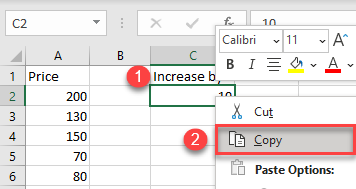1. Select the cells where you want to subtract the value and right-click on the data range (here, A2:A6). In the drop down, click Paste Special.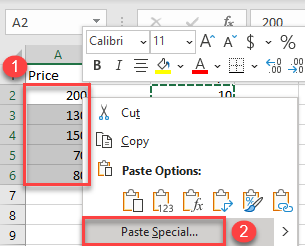1. This brings up the Paste Special dialog box. Under the Operation section choose Add, and then click OK.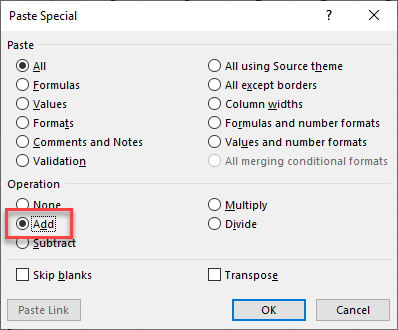You’ll see the result as a number within the same cells. (Here, 10 was added to each value from the data range A2:A6.) This method is better if you don’t want to display the results in a new column and don’t need to retain a record of the original values.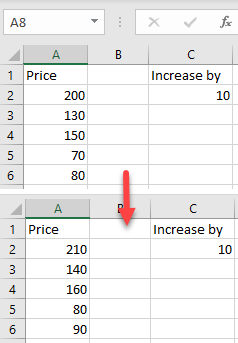## Add Column With Cell References

To add an entire column to another using cell references, select the cell where you want to display the result, and enter = (equal) and the cell reference for the first number then + (plus) and the reference for the cell you want to add.

For this example, calculate the summary of Price 1 (A2) and Price 2 (B2). So, in cell C2, enter:

``=A2+B2``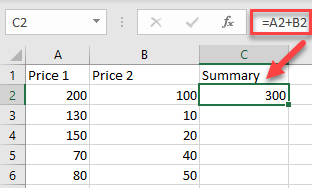To apply the formula to an entire column, just copy the formula down to the rest of the rows in the table (here, C2:C6).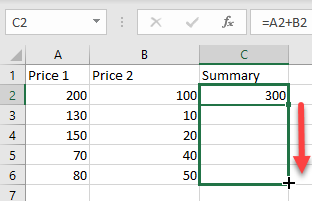Now, you have a new column with the summary.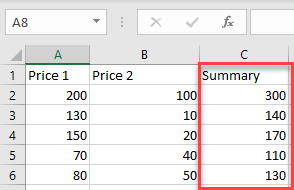Using the methods above, you can also subtract, multiply, or divide cells and columns in Excel.